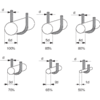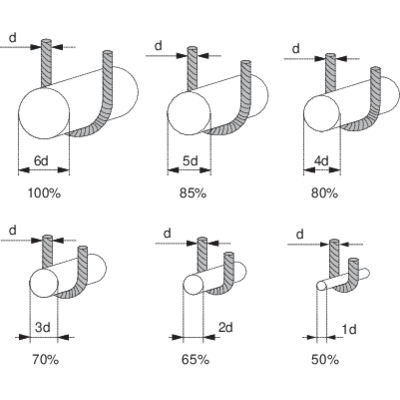## Working load limit (WLL) for steel wire rope sling

Single leg sling
WLL (ton) = (Fmin x KT) / (Zp x g)

Endless sling (choke)
WLL (ton) = (Fmin x KT x 2 x 0,8) / (Zp x g)

Multi-leg sling
WLL (ton) = (Fmin x KT x KL) / (Zp x g)

Fmin = the minimum breaking force of the rope, in kN.
KT = factor for the efficiency of the termination, for ferrule 0,9 and for spliced 0,8.
KL = the leg factor relating to the number of legs and the angle to the vertical, see WLL-table.
Zp = the coefficient of utilization (working coefficient), with the value 5.
g = 9,81

### Bending diameters effect on the working load limit (WLL)

CERTEX recommend that consideration will be taken to that the slings capacity will be lower when the bending diameter decreasing. If, for example the rope bends around a bearing point witch have the same diameter as the rope itself the capacity will decrease to the half, see figure.